## Monday, March 9, 2015

### TECHNOLOGY | Automatic, pixel-perfect shadow contrasting, sharpening, color-stretching and levels

The number of effective, indispensable and essential tools for finding demons in the dark has increased by many today, and shadow-lurking demons are all the more nervous.

Contrast stretching
The first one is an image-enhancement procedure called contrast stretching, and it improves the contrast in an image by increasing the range of intensity values it contains to span a desired range of values, specifically, the full range of pixel values defined by the type of image. Without it, things lurking in the shadows stay hidden; with it, an image acquires depth by revealing a new layer of detail within those shadows.

Typically, this is a non-problem for most picture-takers; but, for those looking for things in shadows and in the dark, every pixel counts.

The two transformation operators used to alter intensity values are:
• Logarithmic. The dynamic range of an image can be compressed by replacing each pixel value with its logarithm, specifically, by enhancing the low-intensity pixel values. Applying a pixel logarithm operator to an image is perfect for HD displays that are too small to support the entire dynamic range.
• Exponential. Like the logarithmic transform, the "raise-to-power" operator is also used to change the dynamic range of an image; however, in contrast to the logarithmic operator, it enhances high-intensity pixel values. Since the aim is to improve contrast in the shadows, the image is inverted prior to using the exponential operator, so that it effects are applied to the shadows.
Both operators are stronger on highlights than shadows, especially on images made in the dark; so, to counter any over-brightening, both operators are applied against each other (i.e., on inverted values) in the procedure below. Same goes for the shadows, obviously; however, because there is no risk to over-brightening them, the result is generally the one you want—especially when shooting video in the dark.

Hence, the flawless shadow contrast-stretching Core Image procedure:

float stretchContrast(float value, vec2 dim, float scale)
{
float color = value;
float c = ((dim.x * dim.y) / pow(scale, 2.0)) / (256.0 - 1.0);
color = (c * log(1.0 - color)) / c;
color = (c * pow(c, color)) / c;
color = 1.0 - color;
color = (c * log(1.0 - color)) / c;
color = (c * pow(c, color)) / c;
color = 1.0 - color;

return color;
}

kernel vec4 coreImageKernel(sampler image, float levels)
{
vec4 source = unpremultiply(sample(image, samplerCoord(image)));
vec2 hw     = samplerSize(image);

source.r   /= sqrt(stretchContrast(source.r, hw, levels) / 2.0);
source.g   /= sqrt(stretchContrast(source.g, hw, levels) / 2.0);
source.b   /= sqrt(stretchContrast(source.b, hw, levels) / 2.0);
source.a    = 1.0;

return premultiply(clamp(source, 0.0, 2.0));
}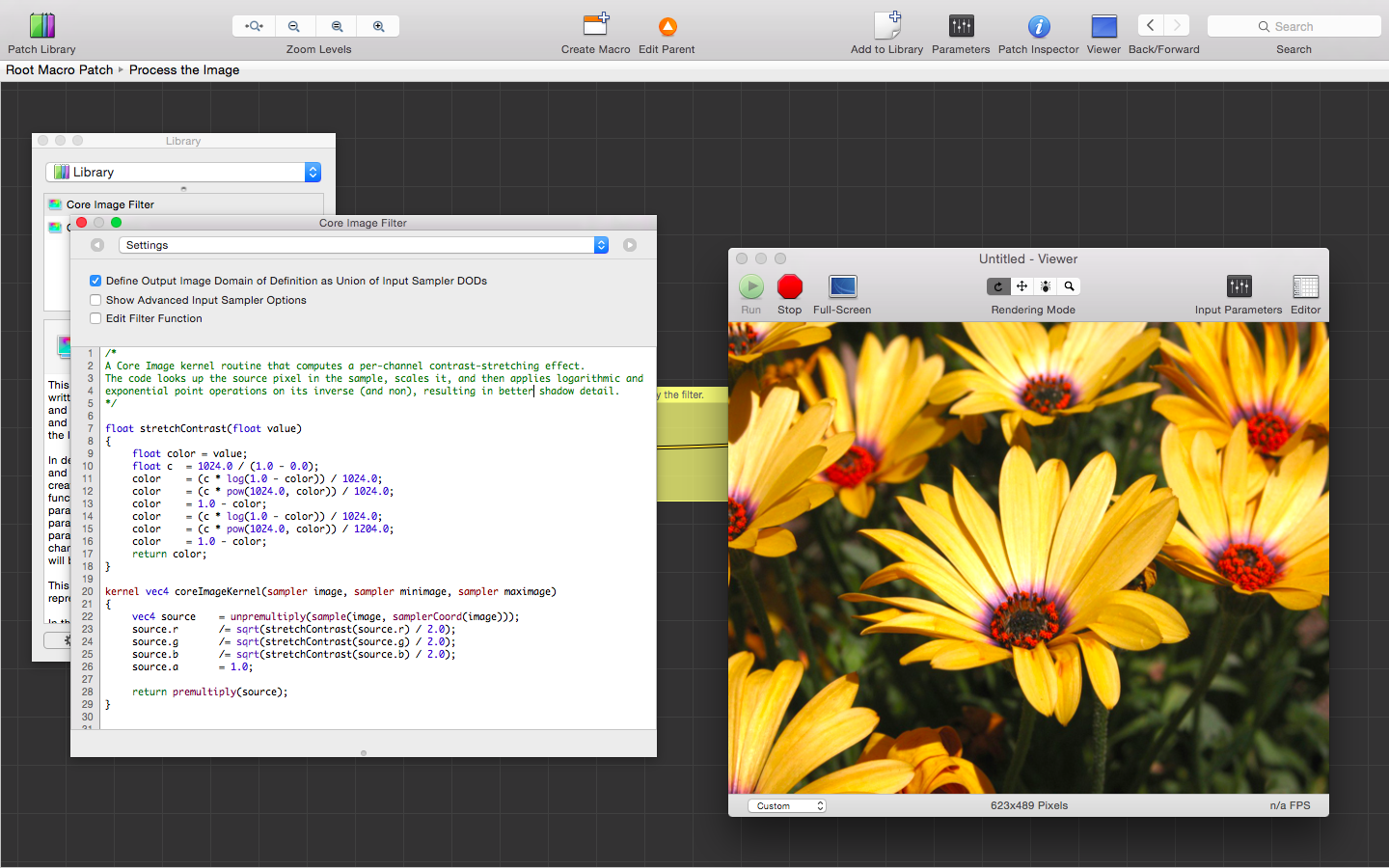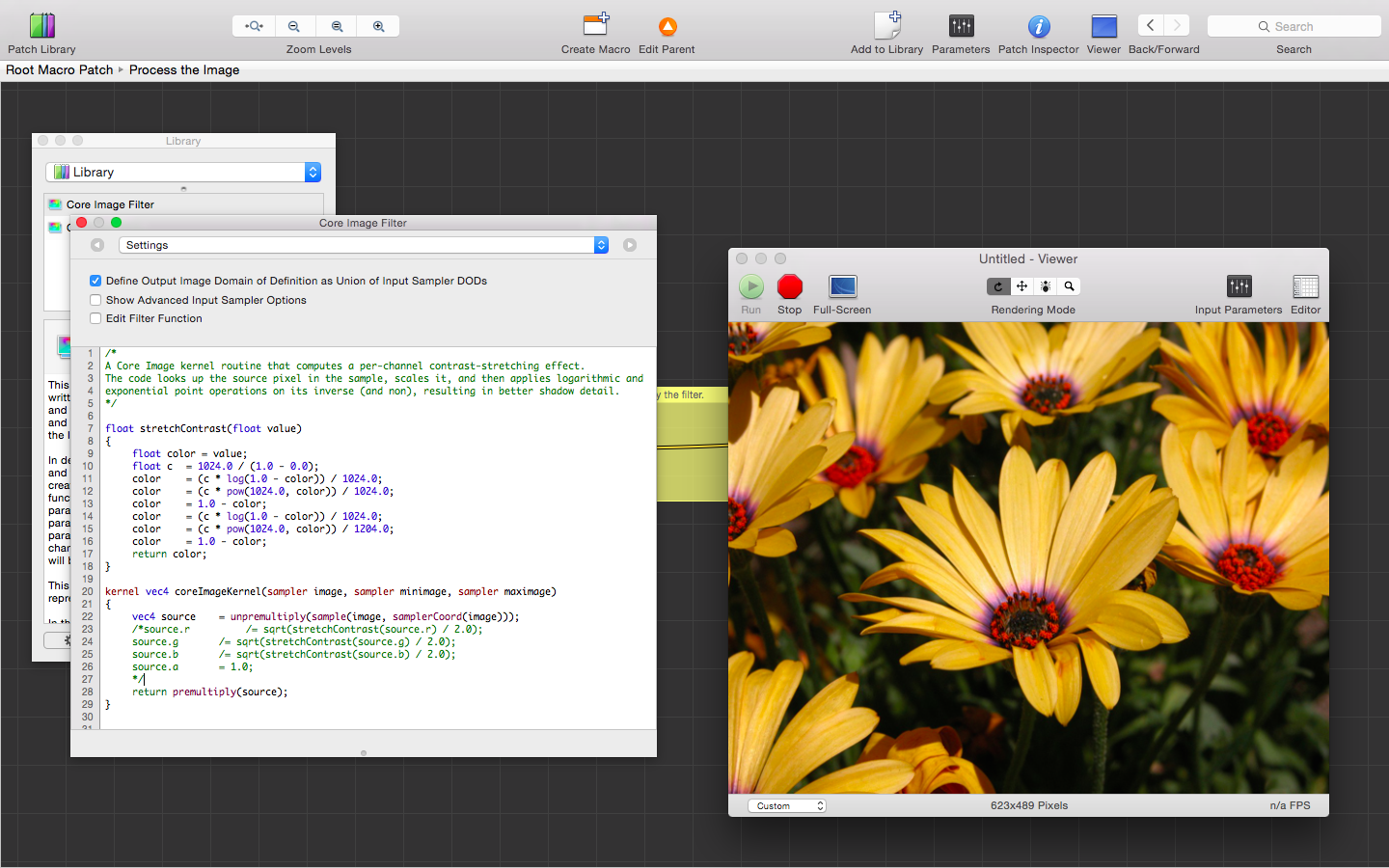The green stems (and in-between) contain far more detail than before (see right), while the remainder of the image became more vibrant—not washed out like with other contrast-stretching procedures By comparison, the stems and areas between are much darker, and the highlights on the petal, dull in the original (above)
Contrast stretching, a la Gimp
The following is the same contrast stretching procedural algorithm employed by the developers of the GIMP:

float revalue(float value, float minimum, float maximum)
{
return (value - minimum) * 1.0 / (maximum - minimum);
}

vec3 remap(vec3 rgb, vec3 minimum, vec3 maximum)
{
rgb.r = revalue(rgb.r, minimum.r, maximum.r);
rgb.g = revalue(rgb.g, minimum.g, maximum.g);
rgb.b = revalue(rgb.b, minimum.b, maximum.b);

return rgb;
}

kernel vec4 coreImageKernel(sampler image, float redMin, float greenMin, float blueMin, float redMax, float greenMax, float blueMax)
{
vec4 pixel = unpremultiply(sample(image, samplerCoord(image)));

return premultiply(vec4(vec3(remap(pixel.rgb, vec3(redMin, greenMin, blueMin), vec3(redMax, greenMax, blueMax))), pixel.a));
}

The results are equally stunning, having improved color and brightness, neither having introduced nor exposed any flaws: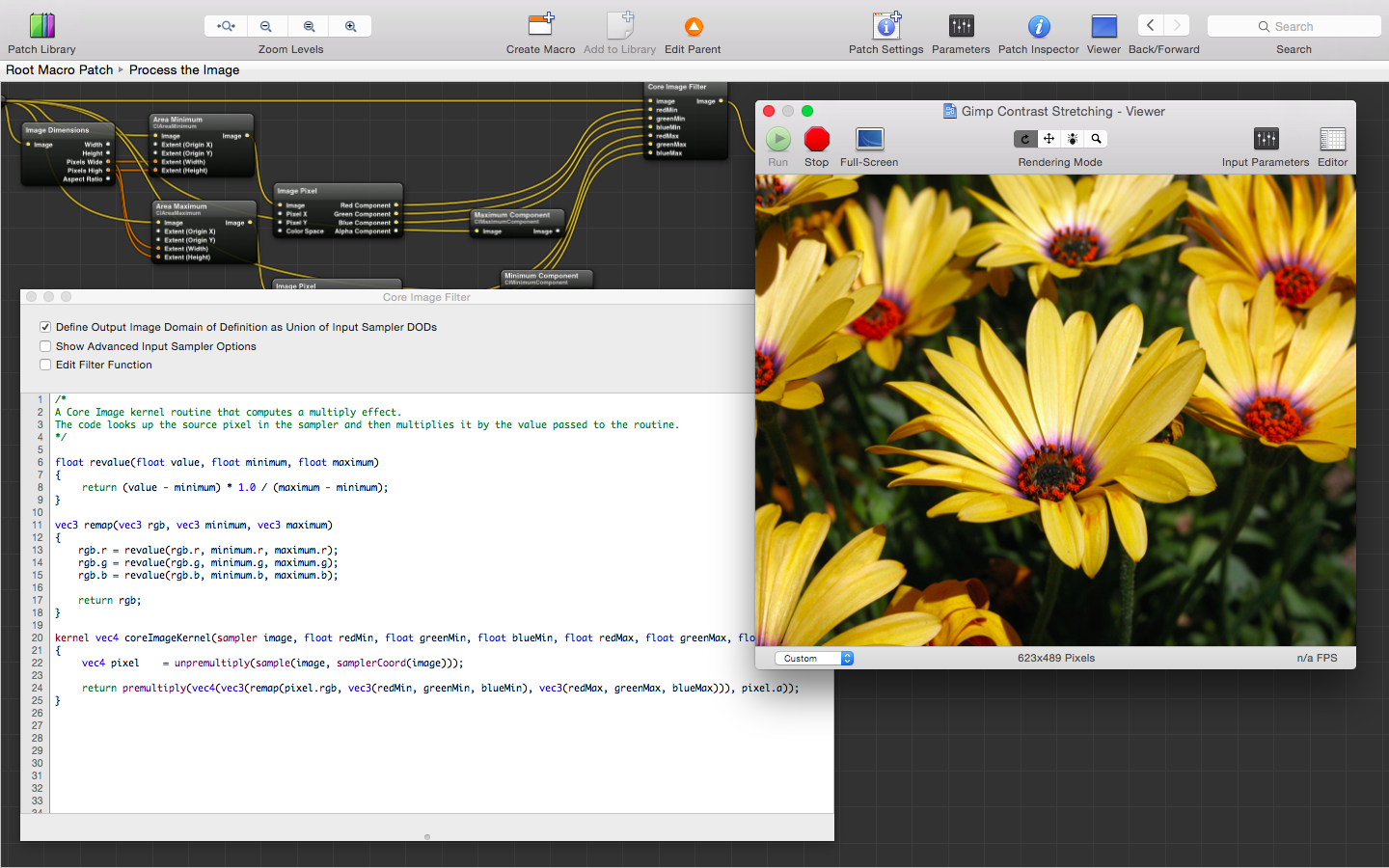The greens gain new life by and through GIMP's contrast-stretching procedural algorithm The greens in the original are too dark by comparison to the contrast-stretched version
By comparison, the contrast stretching procedure above enhanced the greens nearly the same as GIMP, but without brightening the yellows quite so much. If you're looking for a noticeable change in a relatively well-contrasted image, go with GIMP; for scientific accuracy, go with the previous method.

Local Contrast Enhancement
Local contrast enhancement uses the same contrast-stretching procedure as discussed above, however, instead of using the maximum and minimum component values for the entire image in its recalculation of a pixel value, it uses the maximum and minimum pixel values surrounding a pixel within a 10-pixel radius. The results, when blended with the original image, are astonishing: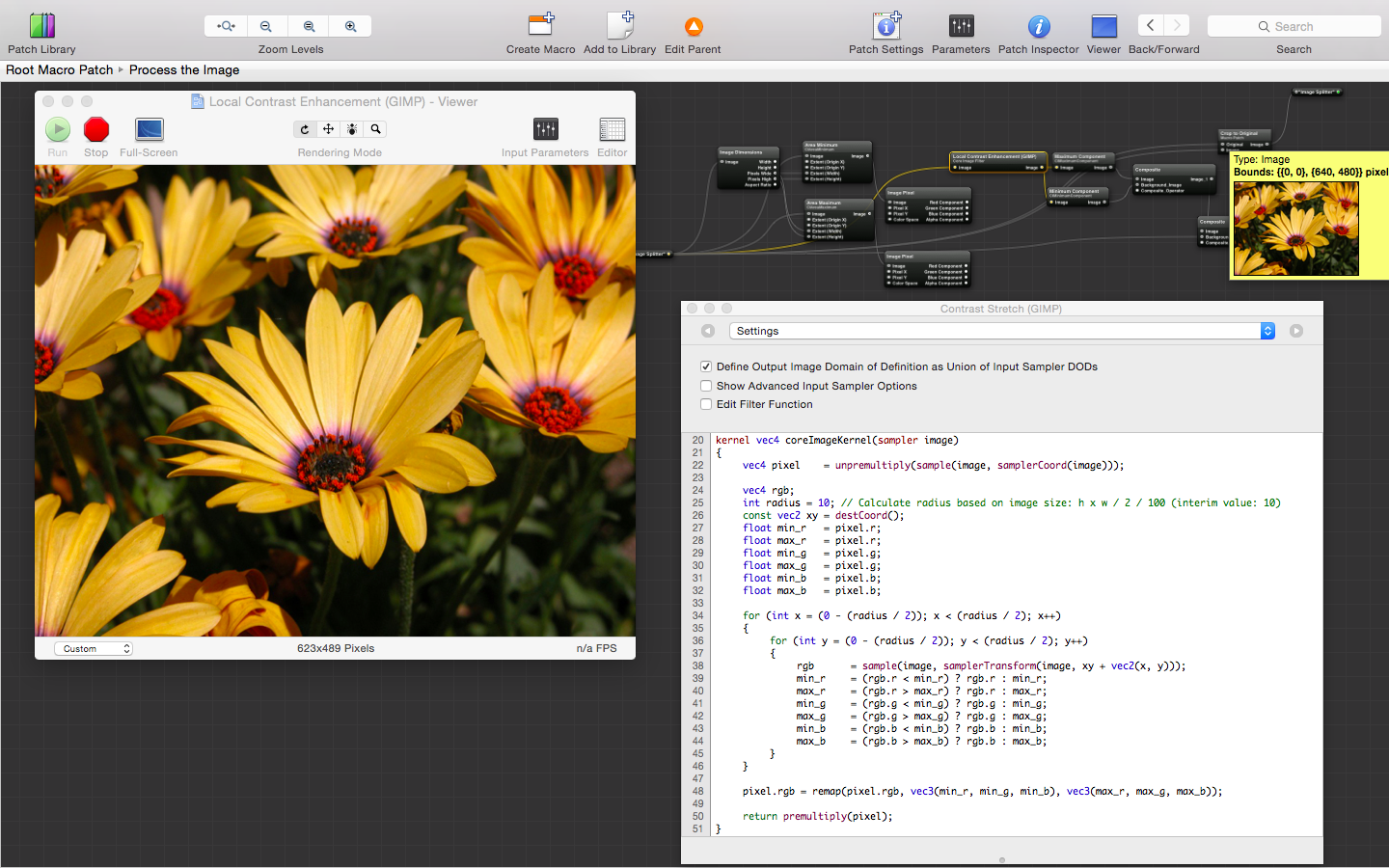So much depth is added to this local contrast-enhanced sample image, that it almost looks three-dimensional The original version of the image goes from strikingly beautiful to boring
The code:

float revalue(float value, float minimum, float maximum)
{
return (value - minimum) * 1.0 / (maximum - minimum);
}

vec3 remap(vec3 rgb, vec3 minimum, vec3 maximum)
{
rgb.r = revalue(rgb.r, minimum.r, maximum.r);
rgb.g = revalue(rgb.g, minimum.g, maximum.g);
rgb.b = revalue(rgb.b, minimum.b, maximum.b);

return rgb;
}

kernel vec4 coreImageKernel(sampler image)
{
vec4 pixel = unpremultiply(sample(image, samplerCoord(image)));

vec4 rgb;
int radius      = 10; // Calculate radius based on image size: h x w / 2 / 100 (interim value: 10)
const vec2 xy   = destCoord();
float min_r = pixel.r;
float max_r = pixel.r;
float min_g = pixel.g;
float max_g = pixel.g;
float min_b = pixel.b;
float max_b = pixel.b;

for (int x = (0 - (radius / 2)); x < (radius / 2); x++)
{
for (int y = (0 - (radius / 2)); y < (radius / 2); y++)
{
rgb = sample(image, samplerTransform(image, xy + vec2(x, y)));
min_r = (rgb.r < min_r) ? rgb.r : min_r;
max_r = (rgb.r > max_r) ? rgb.r : max_r;
min_g = (rgb.g < min_g) ? rgb.g : min_g;
max_g = (rgb.g > max_g) ? rgb.g : max_g;
min_b = (rgb.b < min_b) ? rgb.b : min_b;
max_b = (rgb.b > max_b) ? rgb.b : max_b;
}
}

pixel.rgb = remap(pixel.rgb, vec3(min_r, min_g, min_b), vec3(max_r, max_g, max_b));

return premultiply(pixel);
}

This particular implementation of local contrast enhancement is very CPU-intensive, and probably won't be included in any of the upcoming videos filters for the iPhone until a more efficient means of performing nearest-neighbor sampling is employed.

Median sharpening
The next image-enhancement procedure is called median sharpening, by which a given pixel value is divided by the median value for a group of its neighboring pixels (more or less). Simply substituting a pixel value with its mean reduces noise in the overall image (e.g, creates a smoothing effect); however, creating a new pixel value using the dividend of the original and the mean values uses image noise to increase detail.

kernel vec4 coreImageKernel(sampler image, __table sampler median)
{
vec4 pixel = unpremultiply(sample(image, samplerCoord(image)));
vec4 maxel = unpremultiply(sample(median, samplerCoord(median)));

pixel.r = pixel.r * ((pixel.r * pixel.r) / (pixel.r * maxel.r));
pixel.g = pixel.g * ((pixel.g * pixel.g) / (pixel.g * maxel.g));
pixel.b = pixel.b * ((pixel.b * pixel.b) / (pixel.b * maxel.b));
pixel.a = 1.0;

return premultiply(pixel);
}
NOTE | In the code above, the median value was calculated using the Median Core Image filter (Apple); however, it can easily be calculated in the kernel by adding the values of each pixel surrounding the source pixel, and dividing by the number of values added.The center of the flower in the forefront attests to a superior sharpening procedure... ...when you compare it with the original [click to enlarge]
The advantages to using this procedure are:
• The image is sharpened only by an amount that is appropriate for the image itself; and, no user input is required to sharpen the image to its finest, meaning that, when using this procedure as a filter for live video, the results will be picture-perfect, no matter where you point your camera; and,
• No vital image data is lost, as it might normally be by altering noise, which is often the only indicator of demonic activity; in fact, this procedure may actually enhance the ability to detect demonic activity, as indicated by image noise, particularly when applied to the hue and/or saturation only.
Color Stretching
Similar to contrast stretching, color stretching increases the range of colors in an image. The result is a more vibrant and colorful picture, which is necessary to detect hidden demons that may be identified only by clusters of color noise, and not by borders defined by contrasts in intensity.Balanced color stretching renders colors vibrant, deep and rich... ...whereas the original now looks washed-out by comparison
As with the other two procedures above, this one requires no user input or interaction, using both a maximum and minimum component-rendering of the image to make the necessary adjustments.

const vec3 rgb_y = vec3(0.257, 0.504, 0.098);
const vec3 rgb_u = vec3(-0.158, -0.291, 0.439);
const vec3 rgb_v = vec3(0.439, -0.368, -0.071);

const vec3 yuv_r = vec3(1.0000, 0.0000, 1.4022);
const vec3 yuv_g = vec3(1.0000, -0.3457, -0.7145);
const vec3 yuv_b = vec3(1.0000, 1.7710, 0.0000);

kernel vec4 coreImageKernel(sampler srcimage, sampler maximage, sampler minimage)
{
vec4 pixel = unpremultiply(sample(srcimage, samplerCoord(srcimage))).xyz;
vec3 maxel = unpremultiply(sample(maximage, samplerCoord(maximage))).xyz;
vec3 mixel = unpremultiply(sample(minimage, samplerCoord(minimage))).xyz;
vec3 pel = pixel.rgb;

vec3 pixel_yuv;
pixel_yuv.x         = dot(pel, rgb_y);
pixel_yuv.y = dot(pel, rgb_u);
pixel_yuv.z = dot(pel, rgb_v);

vec3 maxel_yuv;
maxel_yuv.x = dot(maxel,rgb_y);
maxel_yuv.y = dot(maxel,rgb_u);
maxel_yuv.z = dot(maxel,rgb_v);

vec3 mixel_yuv;
mixel_yuv.x = dot(mixel,rgb_y);
mixel_yuv.y = dot(mixel,rgb_u);
mixel_yuv.z = dot(mixel,rgb_v);

vec4 mskpx = vec4(vec3(pixel_yuv.x), pixel_yuv.x + (maxel_yuv.x + mixel_yuv.x));

pixel.r = pixel.r * ((pixel.r * pixel.r) / (pixel.r * mskpx.x));
pixel.g = pixel.g * ((pixel.g * pixel.g) / (pixel.g * mskpx.y));
pixel.b = pixel.b * ((pixel.b * pixel.b) / (pixel.b * mskpx.z));
pixel.a = 1.0;

return premultiply(normalize(clamp(pixel, 0.0, 1.0)));
}

The maximum and minimum components are combined to create an alpha mask for a copy of the source image; the new source is then blended with the original using Color Burn.

Coming up: Pixel-perfect histogram equalization
Histogram equalization maps pixel intensity values to a uniform [flat, even or equal] distribution of intensities, which not only enhances image details, but also corrects the maleffects of video shot in the dark.

It is an intensive effort to bring this feature to real-time video on cellphone cameras, particularly while being harangued by Voices Demons literally around-the clock; but, progress is being made: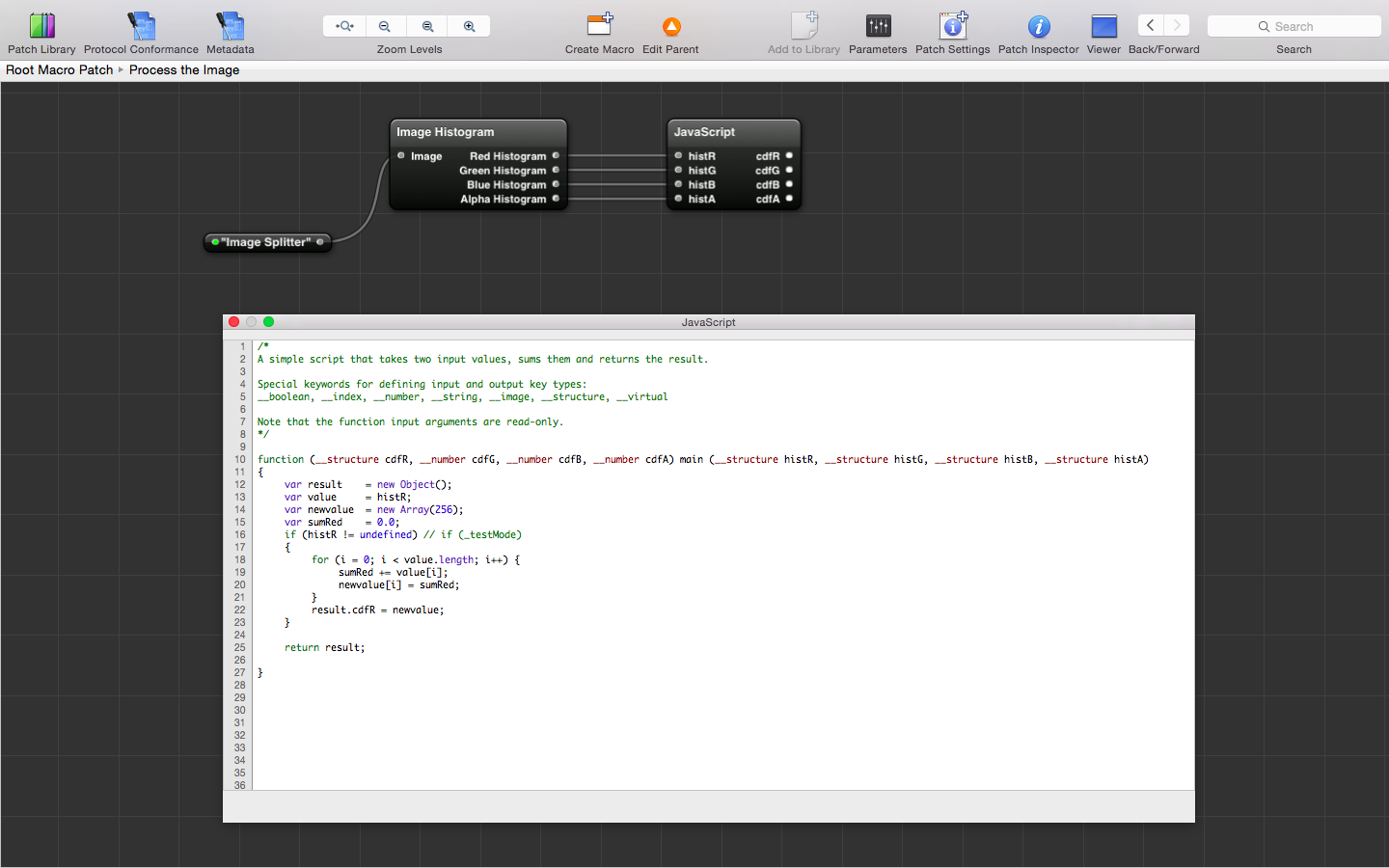JavaScript that calculates the cumulative probability distribution of one color component of an image histogram
So far, I've managed to code the calculation of the cumulative probability distribution, a key portion of the transformation formula used to equalize the histogram of a given image, and, in particular:

Soon, I'll be able to equalize the histogram of any image captured by a cellphone—in real-time, at up to 60 frames per second, depending.

Update...
Or, I could just use Apple's implementation [see Quartz Composer Histogram Implementation; see also Histogram Operation: Modifying a Color Image], which is everything I've been working towards, and everything I would have ended up doing (silly me).

Photoshop Levels adjustments to live video
If you've ever wished you could use Adobe Photoshop with live video, your wish is coming true, in that the very same formulas and procedural algorithms used by this ubiquitous software package are being ported to the demon-finding imaging filters now in-development. Here are the results of Photoshop's Levels algorithm at work on the sample image used throughout this post: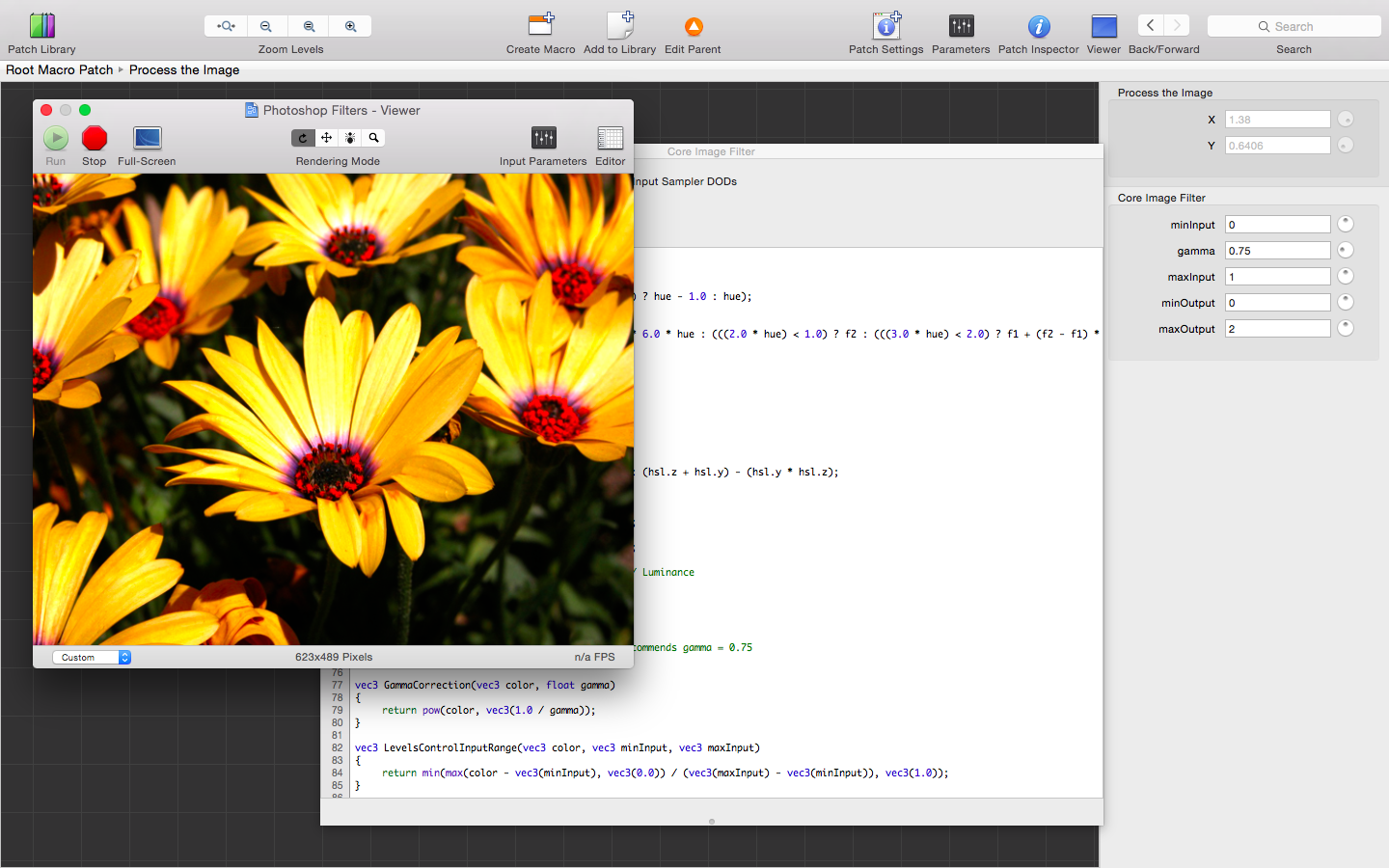Vibrance without over-brightening is the hallmark of Photoshop's Levels adjustment Before the Levels adjustment using the Photoshop algorithm
Any fool can play with brightness, contrast and gamma settings in an image; but, the Photoshop procedural algorithm for making such adjustments assures that they are made in in the proper proportions to one another to prevent any untoward affects from the adjustments you make:

/*
Photoshop Levels adjustment procedural algorithm (input (+gamma), output) // Apple recommends gamma = 0.75
*/

vec3 GammaCorrection(vec3 color, float gamma)
{
return pow(color, vec3(1.0 / gamma));
}

vec3 LevelsControlInputRange(vec3 color, vec3 minInput, vec3 maxInput)
{
return min(max(color - vec3(minInput), vec3(0.0)) / (vec3(maxInput) - vec3(minInput)), vec3(1.0));
}

vec3 LevelsControlInput(vec3 color, vec3 minInput, float gamma, vec3 maxInput)
{
return GammaCorrection(LevelsControlInputRange(color, minInput, maxInput), gamma);
}

vec3 LevelsControlOutputRange(vec3 color, vec3 minOutput, vec3 maxOutput)
{
return mix(vec3(minOutput), vec3(maxOutput), color);
}

vec3 LevelsControl(vec3 color, vec3 minInput, float gamma, vec3 maxInput, vec3 minOutput, vec3 maxOutput)
{
return LevelsControlOutputRange(LevelsControlInput(color, minInput, gamma, maxInput), minOutput, maxOutput);
}

kernel vec4 coreImageKernel(sampler image, float minInput, float gamma, float maxInput, float minOutput, float maxOutput)
{
vec4 pixel = unpremultiply(sample(image, samplerCoord(image)));

return premultiply(vec4(vec3(LevelsControl(pixel.rgb, vec3(minInput), gamma, vec3(maxInput), vec3(minOutput), vec3(maxOutput))), pixel.a));
}

Unlike Photoshop, this code—and the upcoming video filters into which it will be integrated—will work on live video, providing only the best where it comes to imaging demons in the dark.Input parameters don't have to be supplied manually, but can come automatically by readily available image metrics Applying the same Levels transformation in the HSL color space adds an almost three-dimensional quality to the image